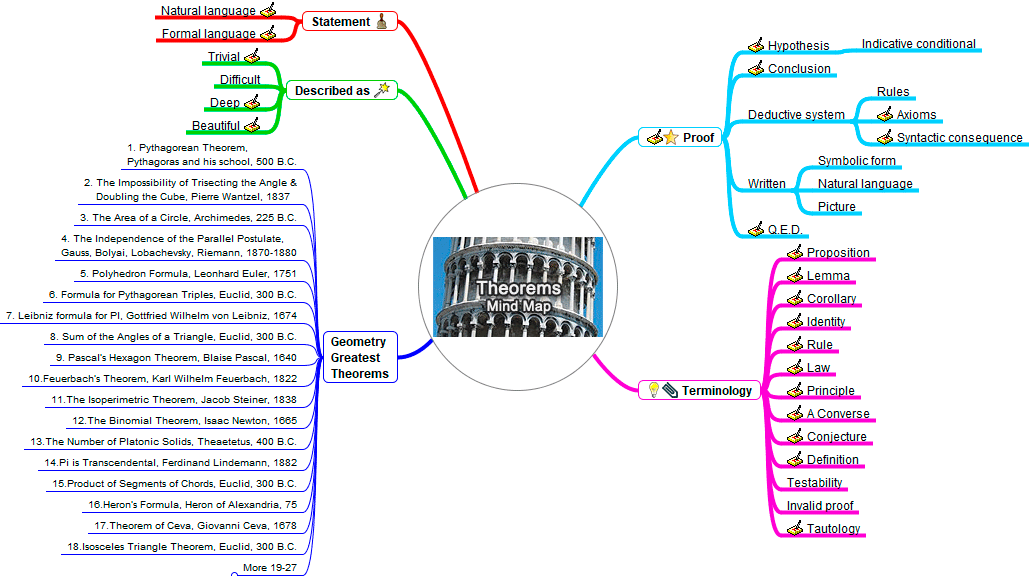# Mind Map: TheoremsTheorem
In mathematics, a theorem is a statement, often stated in natural language, that can be proved on the basis of explicitly stated or previously agreed assumptions.

The proofs of theorems have two components, called the hypotheses and the conclusions. The proof of a mathematical theorem is a logical argument demonstrating that the conclusions are a necessary consequence of the hypotheses, in the sense that if the hypotheses are true then the conclusions must also be true, without any further assumptions.

Mind Map
The mind map above is an image-centered diagram that represents connections between various topics and concepts related to math theorems.Home | Geometry | Mind Maps | Email | Post a commen